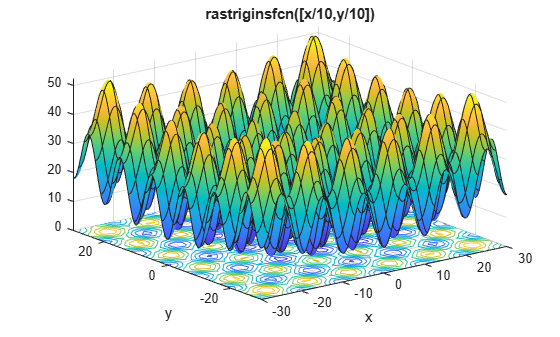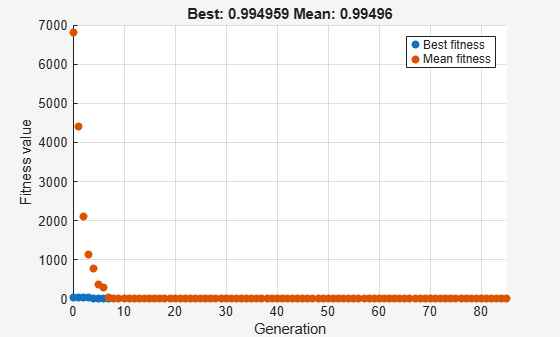Minimize Rastrigins' Function Using ga, Problem-Based

This example shows how to minimize a function with multiple minima using the genetic algorithm in the problem-based approach. For two variables x and y, Rastrigin's function is defined as follows.

ras = @(x, y) 20 + x.^2 + y.^2 - 10*(cos(2*pi*x) + cos(2*pi*y));

Plot the function scaled by 10 in each direction.

rf3 = @(x, y) ras(x/10, y/10);
fsurf(rf3,[-30 30],"ShowContours","on")
title("rastriginsfcn([x/10,y/10])")
xlabel("x")
ylabel("y")The function has many local minima and a global minimum value of 0 that is attained at x = 0, y = 0. See What Is Global Optimization?

Create optimization variables x and y. Specify that the variables are bounded by $±100$.

x = optimvar("x","LowerBound",-100,"UpperBound",100);
y = optimvar("y","LowerBound",-100,"UpperBound",100);

Create an optimization problem with the objective function rastriginsfcn(x).

prob = optimproblem("Objective",ras(x,y));

Note: If you have a nonlinear function that is not composed of polynomials, rational expressions, and elementary functions such as exp, then convert the function to an optimization expression by using fcn2optimexpr. See Convert Nonlinear Function to Optimization Expression and Supported Operations for Optimization Variables and Expressions.

Create ga options to use the gaplotbestf plot function.

options = optimoptions("ga","PlotFcn","gaplotbestf");

Solve the problem using ga as the solver.

rng default % For reproducibility
[sol,fval] = solve(prob,"Solver","ga","Options",options)
Solving problem using ga.
Optimization terminated: average change in the fitness value less than options.FunctionTolerance.sol = struct with fields:
x: 0.9950
y: 0.9950

fval = 1.9899

Is the resulting function value the lowest minimum? Perform the search again. Because ga is a stochastic algorithm, the results can differ.

[sol2,fval2] = solve(prob,"Solver","ga","Options",options)
Solving problem using ga.
Optimization terminated: average change in the fitness value less than options.FunctionTolerance.sol2 = struct with fields:
x: 0.9950
y: -4.9289e-06

fval2 = 0.9950

The second solution is better because it has a lower function value. A solution returned by ga is not guaranteed to be a global solution.